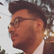# Double Equals vs Triple Equals in JavaScript

March 22, 2019

Unlike other languages JavaScript has two ways to determine equalities. It can be rather confusing for people like myself who come from typed languages like Java or C++. My goal is to explain both == and === in a clear and concise manner through definition and examples.

#### Tripe Equals (strict equality)

The triple equals compares the two values for equality. No extra work is done, it simply checks if the both values have the same type and if they do it then checks whether the two values are the same. If the types are not the same it returns false. If the the types are the same, but the values are different it returns false. Here are some examples:

``````7 === 7     //true
7 === '7'   // false``````

#### Double Equals (loose equality)

The double equals comparison works by first converting the both values to a common type and then undergoing a strict comparison as === does. Let's look at an example to make the explanation a bit more comprehensible. The code below is comparing a Number and a String. The double equals comparison first converts both values to a common type (in this case a Number) and then runs a strict comparison (i.e., it checks if 17 and 17 are the same type and are equal which is true since in the previous step it converted both values to Number).

``````17 == '17' // true
17 == 17   // true``````

#### Closing

It's hard to forget the difference between the two. With that being said, I recommend you write code that allows you to use triple equals rather than double equals. Using double equals can cause unwanted conversions producing inaccurate results.Personal blog by Raul Sanchez